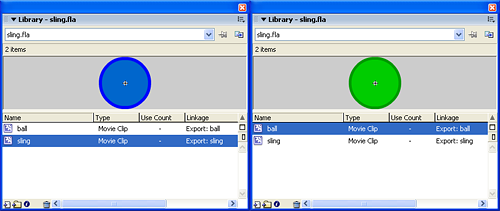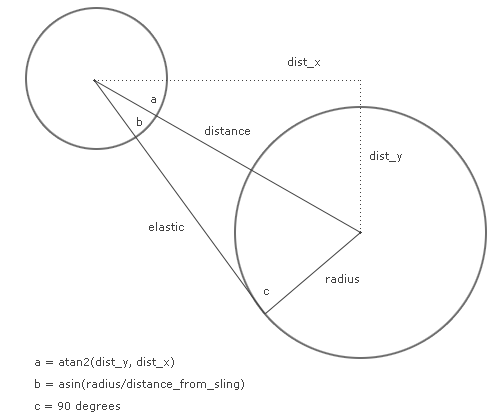# Throw a ball with a sling physics Flash tutorial

August 16th update: 2nd part released by Kevin Ward

Here it comes the first tutorial about some strange ways to use ball physics in a game, as said in the rules of Jay is Games competition. We have to create something original and physics related at the same time.

We’ll learn how to throw a ball with a sling.

In order to throw a ball with a sling, you need a ball and a sling. We can define a sling as an elastic connecting two points.

So I am going to create the ball and the points in Flash in this way:Then, in the first frame, this is the actionscript:

```attachMovie("sling", "sling_1", _root.getNextHighestDepth(), {_x:20, _y:200});
attachMovie("sling", "sling_2", _root.getNextHighestDepth(), {_x:480, _y:200});
attachMovie("ball", "ball", _root.getNextHighestDepth(), {_x:250, _y:100});
_root.createEmptyMovieClip("elastic", _root.getNextHighestDepth());
ball.onPress = function() {
startDrag(this);
};
ball.onRelease = function() {
stopDrag();
};```

Line 1: Placing the first of the two sling nodes in the stage

Line 2: Placing the second sling

Line 3: Placing the ball

Line 4: Creating the empty movieclip where we will draw the elastic

Lines 5-7: Routine to drag the ball

Lines 8-10: Routine to release the ball. You already saw this code in the Creation of the engine behind â€œNodesâ€ game with Flash tutorial.

And in only ten lines we have a draggable ball and a sling without elastic. Time to have an aspirin and make the elastic. Or somethins similar, of course…

Let’s define the basics: we want the elastic to bend if the ball is moved below sling points.

```attachMovie("sling", "sling_1", _root.getNextHighestDepth(), {_x:20, _y:200});
attachMovie("sling", "sling_2", _root.getNextHighestDepth(), {_x:480, _y:200});
attachMovie("ball", "ball", _root.getNextHighestDepth(), {_x:250, _y:100});
_root.createEmptyMovieClip("elastic", _root.getNextHighestDepth());
ball.onPress = function() {
startDrag(this);
};
ball.onRelease = function() {
stopDrag();
};
elastic.onEnterFrame = function() {
this.clear();
this.lineStyle(2, 0x009900);
this.moveTo(sling_1._x, sling_1._y);
if (ball._y>182) {
dist_x = ball._x-sling_1._x;
dist_y = ball._y-sling_1._y;
distance_from_sling = Math.sqrt(dist_x*dist_x+dist_y*dist_y);
elastic_length = Math.sqrt(distance_from_sling*distance_from_sling-18*18);
angle = Math.atan2(dist_y, dist_x)+Math.asin(18/distance_from_sling);
this.lineTo(sling_1._x+elastic_length*Math.cos(angle), sling_1._y+elastic_length*Math.sin(angle));
} else {
this.lineTo(sling_2._x, sling_2._y);
}
this.moveTo(sling_2._x, sling_2._y);
if (ball._y>182) {
dist_x = ball._x-sling_2._x;
dist_y = ball._y-sling_2._y;
distance_from_sling = Math.sqrt(dist_x*dist_x+dist_y*dist_y);
elastic_length = Math.sqrt(distance_from_sling*distance_from_sling-18*18);
angle = Math.atan2(dist_y, dist_x)+Math.asin(18/distance_from_sling)*-1;
this.lineTo(sling_2._x+elastic_length*Math.cos(angle), sling_2._y+elastic_length*Math.sin(angle));
} else {
this.lineTo(sling_2._x, sling_2._y);
}
};```

Line 11: Beginning of the script to be executed at every frame for the elastic object

Line 12: Clearing the movieclip

Line 13: Setting the line style to a green, two pixel thick line

Line 14: Placing the pen on the center of the first sling. If you aren’t familiar with draw commands, read Create a flash draw game like Line Rider or others – part 1 tutorial.

Line 15: Determining if the ball bends the elastic. That 182 means nodes _y position (200) minus ball radius (18), that means that the ball is below sling points

Lines 16-18: Calculating the distance between the center of the ball and the center of the left sling point, using the Pythagorean theorem.

Line 19: Having the distance, using the Pythagorean theorem again to calculate the length of the elasitc, that will touch the ball forming an angle of 90 degrees with the radius. You’ll understand why after reading the next line.

Line 20: Determining the angle between the ball and the left sling point as seen a million times with trigonometry. Refer to Create a flash draw game like Line Rider or others – part 3 tutorial for some trigonometry hints.

But in this case you will notice it’s not the well-known code `Math.atan2(dist_y, dist_x)` but there is another `Math.asin(18/distance_from_sling)`. What is it?

Math.asin(x) returns the arc sine for the number specified in the parameter x, in radians. The arc sine is the inverse of the sine. I have to add the angle due to the arc sine to find a spot along ball circumference where a line long distance_from_sling pixels connects the circumference to the center of the left sling.That’s why we used the Pythagorean theorem to calculate the elastic length and why we involved tha arcsin function.

Line 21: Drawing a line as long as elastic_length with an angle inclination using trigonometry.

Line 23: If the ball is not bending the elastic, just draw a straight line from one sling point to another

Lines 25-35: Same thing for the right sling point

And now we have our elastic bending.

Of course it’s a fake because the elastic does not roll around the ball, but it’s just a game…

You may say there are some lines and controls I can delete, since (as example) being the two sling nodes at the same _y position is not necessary to perform the check twice, but I need to keep them separated at the moment because I plan to make the sling points draggable.

We will need to determine the angle and force of fire, and we’ll see it in another tutorial (coming very soon of course! the competition has begun!), but I think you should have an hint with the next movie, where I simply connected the two “elastic” ends with the center of the ball.

As you can see in the movie (and in the previous picture), the angle between one part of the elastic and the radius going to it is always 90 degress. And that arc that is forming, that seems to grow as much as you draw the ball… do you have the solution? Write it as a comment and you’ll have a post dedicated to your discovery.

Meanwhile, take all the flas and give me FB (feedback)

August 16th update: 2nd part released by Kevin Ward

214 GAME PROTOTYPES EXPLAINED WITH SOURCE CODE
// 1+2=3
// 10000000
// 2 Cars
// 2048
// Avoider
// Ballz
// Block it
// Blockage
// Bloons
// Boids
// Bombuzal
// Breakout
// Bricks
// Columns
// CubesOut
// Dots
// DROP'd
// Dudeski
// Eskiv
// Filler
// Fling
// Globe
// HookPod
// Hundreds
// InkTd
// Iromeku
// Lumines
// Magick
// MagOrMin
// Maze
// Memdot
// Nano War
// Nodes
// o:anquan
// Ononmin
// Pacco
// Phyballs
// Platform
// Poker
// Pool
// Poux
// Pudi
// qomp
// Racing
// Renju
// SameGame
// Security
// Sling
// Slingy
// Sokoban
// Splitter
// Sproing
// Stack
// Stringy
// Sudoku
// Tetris
// Threes
// Toony
// Turn
// TwinSpin
// vvvvvv
// Wordle
// Worms
// Yanga
// Zhed
// zNumbers